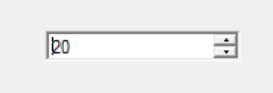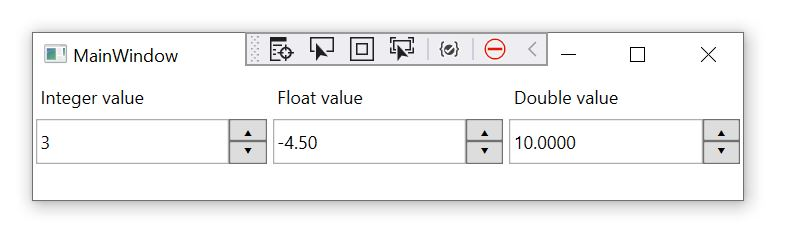Select Page

One of the very useful controls in Windows Form is NumericUPDown control which allows user to enter an integer or decimal number, however this basic control does not exist in WPF standard toolbox and you probably need to install external packages offering it. I have recently created a very basic instance of this control in WPF. Below is an example of the usage.The control is a generic type which requires a specific object to be set in its DATA property which controls various accepts of it. Here is a simple code showing how to set and get the value.
```public partial class MainWindow : Window
{
public MainWindow()
{
InitializeComponent();
// setting an integer number
integerControl.DATA = new IntegerNumber(3, -50, 50);
// setting a float number
floatControl.DATA = new FloatNumber(-4.5f, -25, 34.5f, 0.5f, 2);
// setting a double number
doubleControl.DATA = new DoubleNumber(10, -23.5, 100.00083, 2.5, 4);
}
}```
The parameters from the left to right control the default value , the minimum , the maximum , the size of the increment and the number of decimals. And below is the XML file.
```<Grid Name="grid">
<Grid.ColumnDefinitions>
<ColumnDefinition Width="1*"></ColumnDefinition>
<ColumnDefinition Width="1*"></ColumnDefinition>
<ColumnDefinition Width="1*"></ColumnDefinition>
</Grid.ColumnDefinitions>
<StackPanel Grid.Column="0">
<Label>Integer value</Label>
<uc:IntegerNumericControl x:Name="integerControl" Height="30" Margin="2"
VerticalAlignment="Top" ></uc:IntegerNumericControl>
</StackPanel>
<StackPanel Grid.Column="1">
<Label>Float value</Label>
<uc:FloatNumericControl x:Name="floatControl" Grid.Column="1"  Height="30" Margin="2">
</uc:FloatNumericControl>
</StackPanel>
<StackPanel Grid.Column="2">
<Label>Double value</Label>
<uc:DoubleNumericControl x:Name="doubleControl" Grid.Column="2" Height="30" Margin="2">
</uc:DoubleNumericControl>
</StackPanel>
</Grid>```